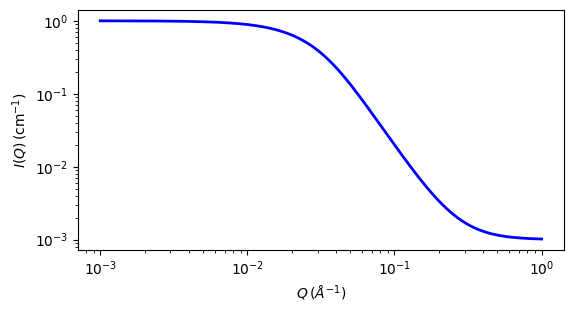# polymer_excl_volume

Polymer Excluded Volume model

Parameter

Description

Units

Default value

scale

Scale factor or Volume fraction

None

1

background

Source background

cm-1

0.001

rg

60

porod_exp

Porod exponent

None

3

The returned value is scaled to units of cm-1 sr-1, absolute scale.

This model describes the scattering from polymer chains subject to excluded volume effects and has been used as a template for describing mass fractals.

Definition

The form factor was originally presented in the following integral form (Benoit, 1957)

$P(Q)=2\int_0^{1}dx(1-x)exp\left[-\frac{Q^2a^2}{6}n^{2v}x^{2v}\right]$

where $$\nu$$ is the excluded volume parameter (which is related to the Porod exponent $$m$$ as $$\nu=1/m$$ ), $$a$$ is the statistical segment length of the polymer chain, and $$n$$ is the degree of polymerization.

This integral was put into an almost analytical form as follows (Hammouda, 1993)

$P(Q)=\frac{1}{\nu U^{1/2\nu}} \left\{ \gamma\left(\frac{1}{2\nu},U\right) - \frac{1}{U^{1/2\nu}}\gamma\left(\frac{1}{\nu},U\right) \right\}$

and later recast as (for example, Hore, 2013; Hammouda & Kim, 2017)

$P(Q)=\frac{1}{\nu U^{1/2\nu}}\gamma\left(\frac{1}{2\nu},U\right) - \frac{1}{\nu U^{1/\nu}}\gamma\left(\frac{1}{\nu},U\right)$

where $$\gamma(x,U)$$ is the incomplete gamma function

$\gamma(x,U)=\int_0^{U}dt\ \exp(-t)t^{x-1}$

and the variable $$U$$ is given in terms of the scattering vector $$Q$$ as

$U=\frac{Q^2a^2n^{2\nu}}{6} = \frac{Q^2R_{g}^2(2\nu+1)(2\nu+2)}{6}$

The two analytic forms are equivalent. In the 1993 paper

$\frac{1}{\nu U^{1/2\nu}}$

has been factored out.

SasView implements the 1993 expression.

The square of the radius-of-gyration is defined as

$R_{g}^2 = \frac{a^2n^{2\nu}}{(2\nu+1)(2\nu+2)}$

Note

This model applies only in the mass fractal range (ie, $$5/3<=m<=3$$) and does not apply to surface fractals ($$3<m<=4$$). It also does not reproduce the rigid rod limit (m=1) because it assumes chain flexibility from the outset. It may cover a portion of the semi-flexible chain range ($$1<m<5/3$$).

A low-Q expansion yields the Guinier form and a high-Q expansion yields the Porod form which is given by

$P(Q\rightarrow \infty) = \frac{1}{\nu U^{1/2\nu}}\Gamma\left( \frac{1}{2\nu}\right) - \frac{1}{\nu U^{1/\nu}}\Gamma\left( \frac{1}{\nu}\right)$

Here $$\Gamma(x) = \gamma(x,\infty)$$ is the gamma function.

The asymptotic limit is dominated by the first term

$P(Q\rightarrow \infty) \sim \frac{1}{\nu U^{1/2\nu}}\Gamma\left(\frac{1}{2\nu}\right) = \frac{m}{\left(QR_{g}\right)^m} \left[\frac{6}{(2\nu +1)(2\nu +2)} \right]^{m/2} \Gamma (m/2)$

The special case when $$\nu=0.5$$ (or $$m=1/\nu=2$$ ) corresponds to Gaussian chains for which the form factor is given by the familiar Debye function.

$P(Q) = \frac{2}{Q^4R_{g}^4} \left[\exp(-Q^2R_{g}^2) - 1 + Q^2R_{g}^2 \right]$

For 2D data: The 2D scattering intensity is calculated in the same way as 1D, where the $$q$$ vector is defined as

$q = \sqrt{q_x^2 + q_y^2}$Fig. 115 1D plot corresponding to the default parameters of the model.

Source

polymer_excl_volume.py

References

1. H Benoit, Comptes Rendus, 245 (1957) 2244-2247

2. B Hammouda, SANS from Homogeneous Polymer Mixtures - A Unified Overview, Advances in Polym. Sci. 106 (1993) 87-133

3. M Hore et al, Co-Nonsolvency of Poly(N-isopropylacrylamide) in Deuterated Water/Ethanol Mixtures, Macromolecules 46 (2013) 7894-7901

4. B Hammouda & M-H Kim, The empirical core-chain model, Journal of Molecular Liquids 247 (2017) 434-440

Authorship and Verification

• Author: